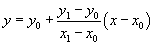←Previous Entry Next Entry→ August 24, 2005 Section 3-14 of Hearn/Baker is titled, Fill-Area Primitives.  These are almost as essential to rendering graphics as points and lines.  It is, of course, a standard, built-in routine for OpenGL, but I want to examine how it works, particularly for simple polygonal areas.  These are "simple" because they are planar and there are no edge-crossings.  However, when you're working in 3D, it may happen that the vertices to define a polygon are not, in fact, planar.  Simple rounding errors can cause this, but you may be working with points on curved surfaces too.   Often, simply dissecting the polygon into triangles can solve the problem (since triangles are always planar,) but in the cases where that won't do, methods have been methodically divined (or devised) as needed.  Let's see. A polygon is convex if all interior angles are less than 180 degrees.  This turns out to be equivalent (under Euclidean assumptions) to saying that every point on the interior can be connected to every other point on the interior by a straight line that lies entirely within the interior.  H/B claim that to be more robust, a graphics package could reject polygons whose vertices are colinear. Given a set of planar polygonal vertices in counterclockwise order, how can it be determined whether it be convex or, the opposite, concave?  Hearn/Baker suggests doing vector cross products for edge vectors emanating from each vertex.  For a convex polygon, all these cross products will be the same sign.  Alternatively, you can use the idea that all vertices of a convex polygon will be on the same side of any give edge. Having established that a set of ordered vertices defines a concave polygon, you can divide it into a set of convex polygons.  Again, edge vector cross products can do this, or, vertex positions relative to some extended edge line.  I rode over to the Alkobar for a newspaper with the H/B stuff about splitting concave polygons and inside/outside tests on my mind and problems came to mind: Given three (ordered?) vertices in a bounded convex region, say, a convex region like a unit square or circle, what is the probability that a randomly chosen fourth point (in order?) will (1) form a concave quadrilateral ? or (2) form a simple quadrilateral? One approach is to get an empirical estimate by performing lots of trials and computing the Monte Carlo thingy.  The algorithm I have in mind for computing the empirical probability is to find the average of probabilities that the fourth point forms a convex quadrilateral with a random set of three initial points.  That is, given three arbitrary triangles in a unit square 1. Determine the coordinates 6 points where the extended triangle edges intesect the perimeter of the unit square. 2. Determine the vertices of polygons opposite the the triangle vertices and compute their areas. 3. Compute the ratio of areas where the fourth point will produce a convex polygon to the area of the unit square (1) This ratio is the probability that a randomly chosen fourth point will form a convex polygon.  So, for part 1: first compute an equation for the extended line, sayand then plug in x=0, x=1, y=0 and y=1 to determine where it might intersect the boundary (only two of these will have value second coordinates.)  Assuming a non-zero slope m has been computed, the coordinate pairs to consider are (0, y0–mx0), (x0–y0/m, 0), (1, y0+(1–x0)m), and (x0+(1–y0)/m, 1). To be on the perimeter of the square, computed coordinates must be between 0 and 1. Taking a visual approach, I come up with the following code: Here's the current deal:   // Based on code from OpenGl Primer by Edward Angel #include #include #include #include #include #define NULL 0 int doubleb; //window ids //singleb, //prototypes void display(); void keyIt(unsigned char, int, int); void reshapeIt(int, int); void display(); void quit_menu(int); void drawObjects(GLenum); //////////////////////////////////////// int main(int argc, char** argv) { glutInit(&argc, argv); //create a double buffered window glutInitDisplayMode(GLUT_DOUBLE | GLUT_RGB); doubleb = glutCreateWindow("double buffered"); //initIt(); glutDisplayFunc(display); glutReshapeFunc(reshapeIt); glutIdleFunc(display); //glutMouseFunc(mouse); //Enter event loop glutMainLoop(); } void display() { glClear(GL_COLOR_BUFFER_BIT); drawObjects(GL_RENDER); glutSwapBuffers(); } void drawObjects(GLenum mode) { float t, temp, x, y; float edgePts; for(float i = 0; i < 1.0; i+=0.1) { for(float j = 0; j < 1.0; j+=0.1) { for(int k= 0;k<3;++k) { t[k] = (float) rand()/(10*RAND_MAX)+i; t[k] = (float) rand()/(10*RAND_MAX)+j; } glColor3f(1.0,0.0,0.0); //red glBegin(GL_TRIANGLES); glVertex2fv(t); glVertex2fv(t); glVertex2fv(t); glEnd(); } } // Sort the points in increasing order of x. // Note: this should be tightened up with a bubble sort... if(t